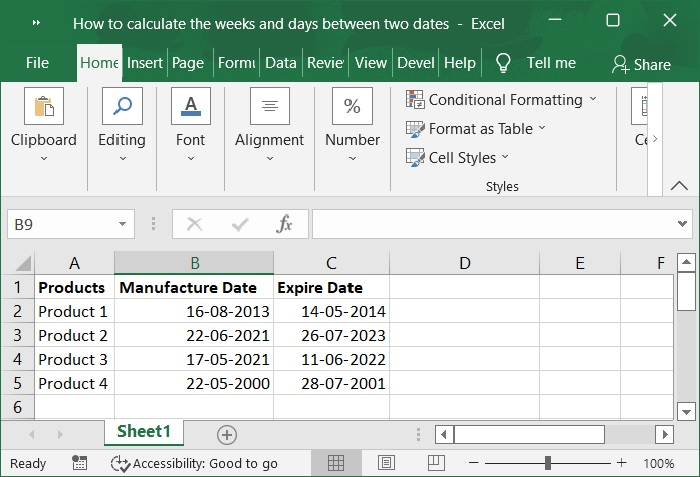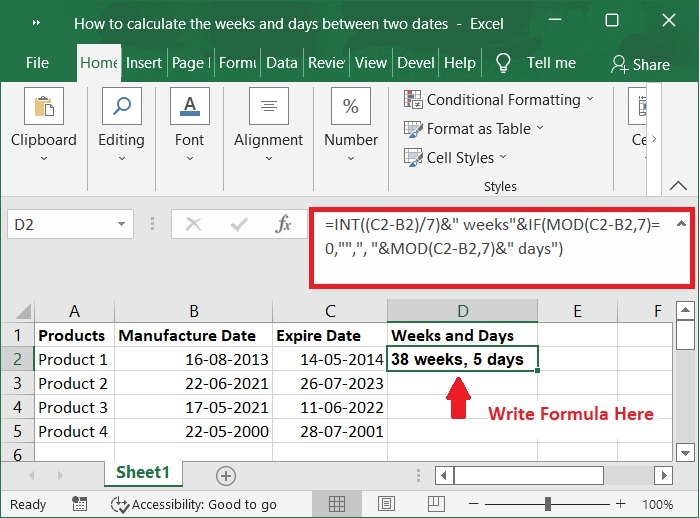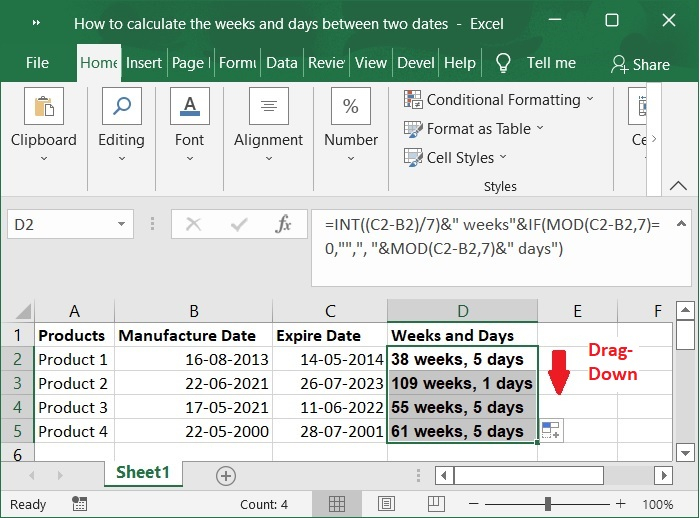# How to calculate the weeks and days between two dates in Excel?

Calculating the number of days, months, years, or weeks that pass between two certain dates may be part of the routine to us. However, have you ever attempted to use Excel to determine the number of weeks and days based on two dates?

In this tutorial, we will use a formula to get the number of weeks and days that exist between any two specific dates. Let’s understand step by step with an example.

## Step 1

In the first step, Let’s assume we have a sample data for columnar format. Please refer to the below screenshot.## Step 2

Now, enter the formula into a cell that is blank, which would be cell D2 in this example. Refer to the below screenshot for same.

=INT((C2-B2)/7)&" weeks"&IF(MOD(C2-B2,7)=0,"",", "&MOD(C2-B2,7)&" days")


Here,

B2 is Manufacture date and C2 is Expired date.## Step 3

Then, to use this formula, simply pull the fill handle to the bottom of its range, and the outcome will be exactly what you require. Please check out the screenshot below.## Points to Note

To calculate date − To determine the total number of days that pass between two dates, simply take the beginning date and deduct it from the ending date. In the event that this spans multiple years, you need to determine the total number of full years. Determine the number of months for the remaining portion of the timeframe. Calculate the number of days for the remaining portion of the period.

To Calculate Weeks − To calculate the number of weeks that have passed since two specific dates, we may apply a straightforward formula: count the number of days that separate the two dates, then divide that number by 7. The result of the formula will be a number with decimal places.

## Conclusion

This tutorial explained how you can use the features in Excel to calculate the weeks and days between two dates.